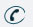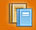781-366-0662

Subject

## Major Areas of Study

Number Sense and Operations : Students will understand numbers, ways of representing numbers, relationships among numbers, and number systems. They will understand the meanings of operations and how they relate to one another, compute fluently and make reasonable estimates.

Topics Covered : Prime Factorization, Greatest Common Factor, Least Common Multiple (Level 1 extensions include scientific notation and absolute value.)

Patterns, Relations, and Algebra : Students will understand patterns, relations, and functions. They will represent and analyze mathematical situations and structures using algebraic symbols, use mathematical models to represent and understand quantitative relationships, and analyze change in various contexts.

Topics Covered : Linear Equations, Systems of Equations, Inequalities, Quadratic Equations, Exponents
(Level 1 extensions include equations of parallel and perpendicular lines, factoring monomials and polynomials, exponential functions, radicals and radical equations, and square root functions.)

Geometry : Students will analyze characteristics and properties of two-and three-dimensional geometric shapes and develop mathematical arguments about geometric relationships. They will specify locations and describe spatial relationships using coordinate geometry and other representational systems, analyze transformation and use symmetry to analyze mathematical situations. In addition, they will use visualization, spatial reasoning and geometric modeling to solve problems.

Topics include : Right Triangle Geometry
(Level 1 extensions include distance and midpoints formulas and topics in trigonometry.)

Data Analysis, Statistics, and Probability : Students will formulate questions that can be addressed with data and collect, organize, and displace relevant data to answer them. They will select and use appropriate statistical methods to analyze data, develop and evaluate inferences and predictions that are based on data, and understand and apply basic concepts of probability.

Topics Covered : Probability, Mean, Median, Mode, Box and Whisker Plots, Standard Deviation
(Level 1 extensions include line of best fit and Venn diagrams.)Subject Classes